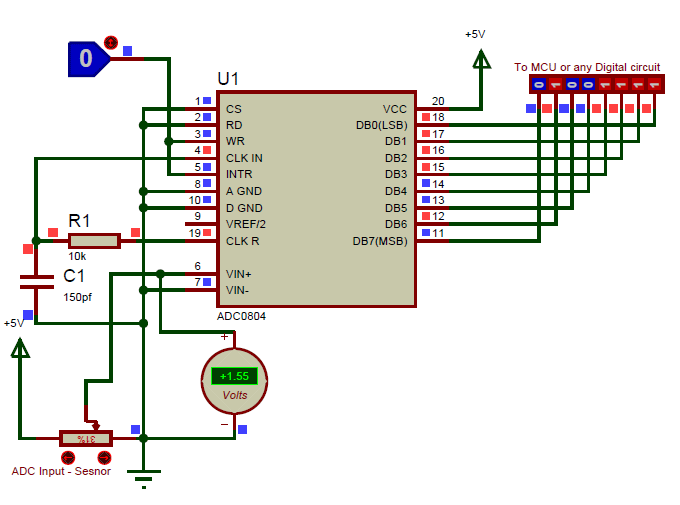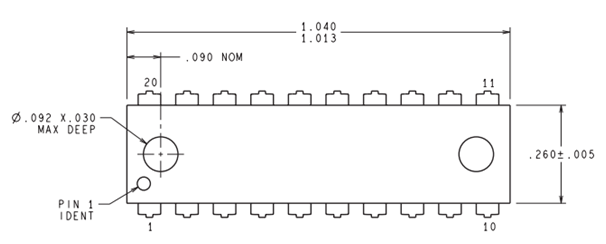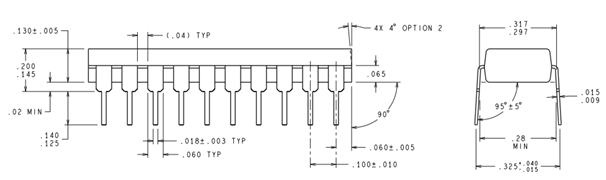[Click the image to enlarge it]

### Features

• Easy to interface with all Microprocessors or works Stand alone.
• Single channel 8-bit ADC module
• On chip Clock available, no need of external Oscillator (Clock)
• Digital output various from 0 to 255
• When Vref = 5V, for every 19.53mV of analog value there will be rise of one bit on digital side (Step size)
• Available in 20-pin PDIP, SOIC packages

Note: Complete Technical Details can be found at the ADC0804 datasheet given at the end of this page.

### Where to use a ADC0804

The ADC0804 is a commonly used ADC module, for projects were an external ADC is required. It is a 20-pin Single channel 8-bit ADC module. Meaning it can measure one ADC value from 0V to 5V and the precision when voltage reference (Vref –pin 9) is +5V is 19.53mV (Step size). That is for every increase of 19.53mV on input side there will be an increase of 1 bit at the output side.

This IC is very Ideal to use with Microprocessors like Raspberry Pi, Beagle bone etc.. Or even to use as a standalone ADC module. Every ADC module requires a clock to function; this IC comes with its own internal clock so you don’t have to worry about it. Hence, if you are looking for a compact ADC module with a decent resolution of 8-bit then this IC is for you.

Since the IC comes with an internal clock we do not need many components to make it work. However to make the internal clock to work we have to use a RC circuit. The IC should be powered by +5V and the both ground pins should be tied to circuit ground. To design the RC circuit simply use a resistor of value 10k and capacitor of 100pf (approx) and connect them to CLK R and CLK IN pins as shown in the circuit below. The chip select (CS) and Read (R) pin should also be grounded. The Vref pin is left free because by default without any connection it will be connected to +5V.

The digital output will be obtained from the pins DB0 to DB7 and the analog voltage should be connected to V in(+) pin as shown in the circuit. Also note that another end of the voltage source (sensor/module) should also be grounded to the circuit for the ADC conversion to work. Now, for the ADC Conversion to start we have o make the Write(WR) pin to go high momentary this can be done connecting the pin to I/O of MPU and toggling it high before every ADC read. Only if this is done the ADC value on the output side will be update.In the above circuit I have used a potentiometer to feed in a variable voltage of 0V to 5V to the Vin pin and the present Voltage is read using a voltmeter. As you can see in the image the voltage value is 1.55V and the resulting binary value is 01001111. Let us see how this binary value can be converted to Analog value, since we will need it while programming/designing.

Binary Value = 01001111

Converting to Decimal  =  (0*128)+(1*64)+(0*32)+(0*16)+(1*8)+(1*4)+(1*2)+(1*1)

=   79

Analog Voltage = Decimal Value * Step size

= 79 * 19.53mV

= 1.54V

The obtained value is 1.54V and the measured voltage is 1.55V which are very much close. So this is how you use an ADC0804 IC.

### Applications

• Operates With Any 8-Bit µP Processors or as a Stand-Alone Device
• Widely used with Raspberry Pi, Beagle Bone and other MPU development platforms
• Interface to Temp Sensors, Voltage Sources, and Transducers

### 2-D Model (PDIP)Component Datasheet